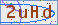# 分辨率测试卡ISO 15739噪点和SNR

SNR = Q = g L /σ

g = d（像素级）/ d L

σ PX =噪点像素（注意：标准偏差σ。相当于RMS噪点）

L=照明或暴露水平 （单位将不会是重要的。）

LF-停止噪点=σFST =σpx/(d(pxl)/d(F-stops))=σpx/(d(pxl)/ d的(log2l))光圈SNR = SNR FST = 1/σFST=(d(pxl)/ d(log2l))/σPX我们将应用方程，d(对数b(x))/dx = 1/(X LN(B))，其中Ln(2)= 0.6931=1/1.4427。现在，从ISO标准，增量增益= gl= d(pxl)/ dl(注线性单位)= d(pxl)/ d(log2 l)·d(log2l)/dl = 1.442(d(pxl)/d(log 2 l))/l。ISO 15739标准的附录D将总信噪比定义为SNR ISO=Lgl /σPX = 1.4427L(d(Pxl)/ d(log2 l))/(LσPX)= 1.4427(d(Pxl)/ d(log2 L))/σPX这就导出：

SNR ISO=1.4427 SNR FST

SNR ISO优于SNR FST的一个因素1.4427，或等价，3.18分贝。文章评论
内容:
验证码: